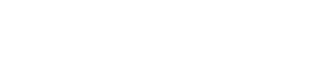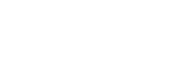# JEE Conic Sections: Hyperbola

Have you ever wondered “What is the shape of the shadow that a household lamp casts on the walls?” From the cooling towers of thermal power plants to the McDonalds logo, you can find hyperbolic shapes in a lot of places around you if only you look for it.

The mathematical concept of Hyperbola is essential for engineering and architectural studies. It’s quite obvious that Hyperbola is very important for JEE. All its properties must be at your fingertips in order to solve the questions asked in JEE.

## Definition

You can think of a hyperbola to be two curves that resemble infinite bows.
A hyperbola is the locus of all points in a plane, the difference of whose distances from two fixed points (focus) is constant.By Ag2gaeh – Own work, CC BY-SA 4.0, Link

Now let me introduce you to the various terms related to hyperbola:By Ag2gaeh – Own work, CC BY-SA 4.0, Link

1) Focus: These are the two fixed points that we discussed in the definition.
2) Center: It is the mid-point of the line segment joining both the foci. It is also the intersection of the transverse and the conjugate axis.
3) Transverse Axis: It is the line joining both the foci. It is also the Major Axis.
4) Conjugate Axis: It is the line perpendicular the Transverse Axis and passes through the center of the hyperbola.
5) Vertex: The point at which the hyperbola intersects the transverse axis. Since the hyperbola intersects at two distinct points, there are two vertices in a hyperbola.

## General Form of Equation of Hyperbola

Ax2 + Bxy + Cy2 + Dx + Ey + F = 0

The expression B2 — 4AC is called the discriminant, which is used to determine the type of conic section.
For a hyperbola, B2 — 4AC > 0.

## Standard Form of Equation of Hyperbola

A hyperbola with Center as Origin (0, 0) and both the foci on the x-axis is given by the equation:By Klaas van Aarsen – Own work, CC BY-SA 3.0, Link

The properties of the above hyperbola are:

1) The center is at O(0, 0).
2) The foci are S(ae, 0) and S1(–ae, 0)
3) The vertices are A(a, 0) and A1(–a, 0)
4) The directrix is given by the equation: x = a/e and x = –a/e
5) The length of the latus rectum is (2b2/a)
6) The length of the transverse axis = 2a
7) The length of the conjugate axis = 2b
8) The distance between 2 focii = 2ae

Now you might be wondering about ‘e’ used in various formulae. ‘e’ is known as the Eccentricity of a conic section. It is the ratio between the focus and the directrix which remains constant for a given conic section. For a hyperbola, e > 1.By Deipnosopher – I created this work entirely by myself., CC BY-SA 3.0, Link

## Focal Distance of a Point

As the name suggests, it is the distance of any arbitrary point on the hyperbola from the focus.
Let P be any point present on the hyperbola,

|S1P — S2P| = 2a

Clearly, the focal distance of any point on the hyperbola is constant and is equal to the length of the Transverse Axis.

## Equation of Hyperbola with (h, k) as CenterThe directions of the axes should be parallel to the co-ordinate axis.

## Parametric Equations

The parametric equations of the general hyperbola being discussed are:

x = a sec θ , y = b tan θ
Or
x = a cosh θ , y = b sinh θ

## Equations of Chord

1) The equation of a chord joining two points P( a sec θ₁ , b tan θ₁) and Q( a sec θ₂ , b tan θ₂) on the hyperbola2) The equation of the chord of the hyperbola bisected at point (x₁, y₁) is given by,

T = S₁

3) Equations of the chord of contact of tangents drawn from a point (x₁, y₁) to the hyperbola is given by,## Equation of Tangent to the Hyperbola

1) In point form the equation of the tangent to the hyperbola is,2) In parametric form the equation of the tangent to the hyperbola is,3) In Slope form the equations of the tangent to the hyperbola is,

y = mx ± √(a²m² — b²)

Here, m is the slope of the normal the tangent to the hyperbola.

4) The line y = mx + c touches the hyperbola if c² = a²m² — b².

## Equation of Normal to the Hyperbola

1) Point Form: In point form the equation of normal to the hyperbola is,2) Parametric Form: The equation of normal to the hyperbola at a point P(a secθ, b tanθ) is,

ax cosθ + by cotθ = a² + b²

3) Slope Form: The equation of normal to the hyperbola is,Here, m is the slope of the normal to the hyperbola being discussed.

4) A maximum of 4 normals can be drawn to a hyperbola from a point P(x₁, y₁)

## Diameter and Conjugate Diameter

1) Diameter: Diameter is the locus of mid-points of all the parallel chords of the hyperbola. The equation of the diameter, bisecting a system of parallel chords with each of slope m to the hyperbola is given by,2) Conjugate Diameter: The diameters of a hyperbola are said to be conjugate if each diameter bisects the chords parallel to the other.
The diameters y = m₁x and y = m₂x are conjugate if,

m₁m₂ = b²/a²

3) In a pair of conjugate diameters of a hyperbola, only one of them meets the hyperbola in real points while the other meets the hyperbola at imaginary points.

### Director CircleBy Ag2gaeh – Own work, CC BY-SA 4.0, Link

The locus of points of intersection of the tangents to the hyperbola, which are perpendicular to each other.

The equation of Director Circle is,

x² + y² = a² — b²

## Rectangular HyperbolaA hyperbola for which the asymptotes are perpendicular is known as a rectangular hyperbola.
In other words, it is a hyperbola with a = b.
Therefore, the eccentricity of a rectangular hyperbola is √2 .

The equation for a rectangular hyperbola can be reduced to,

xy = a²

### Properties

1) Asymptotes are the co-ordinate axes, x = 0 and y = 0.
2) e = √2.
3) Center is O(0, 0).
4) Foci are S(√2c,√2c) and S₁(–√2c, –√2c).
5) Vertices are A(c, c) and A₁(–c, –c).
6) Length of Latus Rectum = 2√2c.
7) In parametric form, the equation is x = ct and y = c/t.

### Summary

1) The point (x₁,y₁) lies outside, on, or inside the hyperbola,2) The combined equation of pair of tangents drawn from an external point P(x₁,y₁)
is SS₁–T².

3) The equation of a chord of the hyperbola whose mid-point is (x₁,y₁) is given by
T = S₁.

4) Eccentricity of a Rectangular Hyperbola is √2 and the angle between asymptotes is 90°.

5) If a triangle is inscribed in a hyperbola, then it’s orthocenter lies on the hyperbola.

In this blog post, you learnt about some important concepts about Hyperbola. Hope you found it useful. For more amazing JEE study material, check out Magoosh JEE Product. Happy learning!